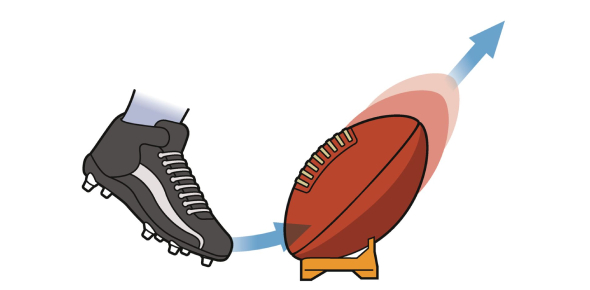# Force And Motion: Basic Test! Quiz

8 Questions | Total Attempts: 439SettingsEver wondered what is the relation between Force and motion? Force is the direct result of motion. The world is full of motion, and each action has some force behind it. With this quiz, you will accumulate information such as inertia, who created the three laws of planetary motion, who created the three laws of motion, whether gravity is an upward force, and what makes gravity happen. This quiz contains the basic facts of force and motion and how they relate to each other.

• 1.
What is inertia?
• A.

A motion in rest/in movement

• B.

A force

• C.

Another word for force

• 2.
What of these is the three laws of motion?
• A.

Law of friction

• B.

Law of inertia

• C.

Law of action and reaction

• D.

Law of heat

• E.

Law of acceleration

• 3.
Who created the three laws of PLANETARY motion?
• A.

Sir Isaac Newton

• B.

Johannes Kepler

• C.

All of the above

• D.

None of the above

• 4.
Who created the three laws of motion?
• 5.
Gravity is upward force.
• A.

True

• B.

False

• 6.
What is the laws of planetary motion?
• 7.
Isaac Newton studied Light.
• A.

True

• B.

False

• 8.
Gravity is like a MAGNET. What makes it happen? Ozone layer or magnetosphere.
• A.

Ozone

• B.

Magnetosphere

Related TopicsBack to top